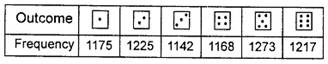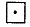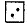### Probability - Test Papers

CBSE Test Paper 01

CH-15 Probability

1. The minimum value of probability is
1. 1
2. $\frac{1}{2}$
3. 0
4. None of these
2. Three coins are tossed together, then the probability of getting at most one head is:-
1. $\frac{1}{8}$
2. $\frac{1}{4}$
3. $\frac{1}{2}$
4. 1
3. Three coins are tossed.The probability of getting 2 heads and 1 tail is
1. $\frac{3}{8}$
2. 1
3. $\frac{2}{3}$
4. $\frac{3}{4}$
4. The sum of the probabilities of all events of a trial is
1. between 0 and 1
2. greater than 1
3. less than 1
4. 1
5. Which of the following cannot be the probability of an event?
1. $\frac{17}{16}$
2. 0.1
3. $\frac{1}{3}$
4. 3$\mathrm{%}$
6. Fill in the blanks:

The sum of all the probabilities of all possible outcomes of an experiment is ________.

7. Fill in the blanks:

The probability of happening of an event always lies from ________ to _________.

8. In tossing a coin 100 times head appears 56 times. What is the probability of head for the coin?

9. Given below is the frequency distribution table regarding the concentration of sulphur dioxide in the air in parts per million of a certain city for 30 days.

 Conc. of SO2 0.00-0.04 0.04-0.08 0.08-0.12 0.12-0.16 0.16-0.20 0.20-0.24 No. of days 4 8 9 2 4 3

Find the probability of concentration of sulphur dioxide in the interval 0.12-0.16 on any of these days.

10. Activity: Note the frequency of two-wheelers, three-wheelers and four-wheelers going past during a time interval, in front of your school gate. Find the probability that any one vehicle out of the total vehicles you have observed is a two-wheeler.

11. Eleven bags of wheat flour, each marked 5kg, actually contained the following weights of flour (in kg): 4.97 5.05 5.08 5.03 5.00 5.06 5.08 4.98 5.04 5.07 5.00Find the probability that any of these bags chosen at random contains more than 5 kg of flour.

12. A bag contains 15 cards bearing numbers 1, 2, 3, 4, ……….. , 14, 15. A card is drawn from the bag. Find the Probability that it bears :
(i) a Prime number
(ii) A number divisible by 2

13. A die is rolled 7200 times. The frequency of each outcome is shown in the table. What is the empirical probability of each outcome?14. An Insurance company selected 2000 drivers at random in a particular city to find a relationship between age and accidents. The data obtained are given in the following table:

 Age of drivers Accidents in one year 0 1 2 3 over 3 18-29 440 160 110 61 35 30-50 505 125 60 22 18 Above 50 360 45 35 15 9

Find the probabilities of the following events for a driver chosen at random from the city:

1. being 18-29 years of age and having exactly 3 accidents in one year.
2. being 30-50 years of age and having one or more accidents in a year.
3. having no accident in one year.
15. An organisation selected 2400 families at random and surveyed them to determine a relationship between income level and the number of vehicles in a family. The information gathered is listed in the table below :

 Monthly income(in Rs.) Vehicles per family 0 1 2 Above 2 Less than 7000 10 160 25 0 7000-10000 0 305 27 2 10000-13000 1 535 29 1 13000-16000 2 469 59 25 16000 or more 1 579 82 88

Suppose a family is chosen. Find the probability that the family chosen is

1. earning Rs. 10000-13000 per month and owning exactly 2 vehicles.
2. earning Rs. 16000 or more per month and owning exactly 1 vehicle.
3. earning less than Rs. 7000 per month and does not own any vehicle.
4. earning Rs. 13000-16000 per month and owning more than 2 vehicles.
5. owning not more than 1 vehicle.

CBSE Test Paper 01
CH-15 Probability

Solution

1. (c) 0
Explanation: Range of probability lies between 0 to 1. So, the minimum value of probability is 0.
2. (c) $\frac{1}{2}$
Explanation: When three coins are tossed simultaneously, the total possible outcomes are {(H,H,H) , (H,H,T) , (H,T,H) , (H,T,T), (T,H,H), (T,H,T), (T,T,H), (T,T,T)}.

The total number of outcomes = 8.

For at most one head, number of heads should be either 0 or 1.
The favourable outcomes are ={(T,H,H), (T,H,T), (T,T,H), (T,T,T)}.
Number of favourable outcomes= 4.

Therefore, the probability of getting at most one head = $\frac{4}{8}$ = $\frac{1}{2}$

3. (a) $\frac{3}{8}$
Explanation: When we toss three coins simultaneously then the possible of outcomes are: (HHH) or (HHT) or (HTH) or (THH) or (HTT) or (THT) or (TTH) or (TTT) respectively; where H is denoted for head and T is denoted for tail.

Therefore, total numbers of possible outcomes are 23 = 8

Events of getting  2 heads and 1 tail = (HHT) ,(HTH) ,(THH) = 3

Therefore, number of favourable outcomes = 3

Therefore,the probability of getting 2 heads and 1 tail = $\frac{3}{8}$

4. (d) 1
Explanation: The first two basic rules of probability are the following:

Rule 1: Any probability P(A) is a number between 0 and 1 (0 < P(A) < 1).

Rule 2: The probability of the sample space S is equal to 1 (P(S) = 1).

5. (a) $\frac{17}{16}$
Explanation: The probability of an event lies between 0 and 1 (or 0-100% expressed in percentage). It cannot be greater than 1 and the value of $\frac{17}{16}$ is greater than 1, so, it cannot be the probability of an event.
6. 1

7. 0, 1

8. P (head) = $\frac{56}{100}$ = 0.56.

9. Total number of days = 30
The probability of concentration of SO2 in the interval 0.12 - 0.16 =  = $\frac{2}{30}$ = 0.06

10. Let you noted the frequency of types of wheelers after school time (i.e. 3 pm to 3.30 pm) for half an hour. Let the following table shows the frequency of wheelers.
Type of wheelers Frequency of wheelers
Two wheelers 125
Three wheelers 45
Four wheelers 30
Probability that a two wheelers passes after this interval = $\frac{125}{200}=\frac{5}{8}$

11. Total number of bags of wheat flour = 11
Number of bags of wheat flour containing more than 5 kg of flour = 7.
∴ Probability that any of the bags, chosen at random, contains more than 5 kg of flour $=\frac{7}{11}$.

12. Total number of cards = 15
(i) Among 1, 2, 3, 4,……., 14,15, prime number are 2, 3, 5, 7, 11, 13
Number of favourable outcomes = 6
P (Prime number) = $\frac{6}{15}=\frac{2}{5}$
(ii) Among 1, 2, 3, 4, ………….., 14, 15
No. divisible by 2 are 2, 4, 6, 8, 10, 12, 14
$\therefore$Number of outcomes = 7
P (no. divisible by 2) = $\frac{7}{15}$

13. Let E1 = event thatcomes, then $P\left({E}_{1}\right)=\frac{1175}{7200}=0.163$
Let E2 = event thatcomes, then $P\left({E}_{2}\right)=\frac{1225}{7200}=0.170$
Similarly, $P\left({E}_{3}\right)=\frac{1142}{7200}=0.159$$P\left({E}_{4}\right)=\frac{1168}{7200}=0.162$
$P\left({E}_{5}\right)=\frac{1273}{7200}=0.177$ and $P\left({E}_{6}\right)=\frac{1217}{7200}=0.169$

14. Total number of drivers = 2000

1. Number of drivers who are 18-29 years old and have exactly 3 accidents in one year is 61
So, P (driver is $18\stackrel{^}{\mathrm{a}}29$ years old with exactly 3 accidents) = $\frac{61}{2000}$
$=0.0305=0.031$
2. Number of drivers having 30-50 years of age and having one or more accidents in one year = 125 + 60 + 22 + 18 = 225
So, P (driver is 30-35 years of age and having one or more accidents)  $\frac{225}{2000}$ =$0.1125=0.113$
3. Number of drivers having no accident in one year 440 + 505 + 360 = 1305
So, P (drivers with no accident) = $\frac{1305}{2000}$ $=0.6525=0.653$
15. Total number of families selected = 2400

1. Number of families earning Rs. 10000-13000 per month and owning exactly 2 vehicles = 29
∴ Probability that the family chosen is earning Rs. 10000-13000 per month and owning exactly 2 vehicles $=\frac{29}{2400}$
2. Number of families earning Rs. 16000 or more per month and owning exactly 1 vehicle = 579
∴ Probability that the family chosen is earning Rs.16000 or more per month and owning exactly 1 vehicle
$=\frac{579}{2400}=\frac{193}{800}$.
3. Number of families earning less than Rs.7000 per month and does not own any vehicle = 10
∴ Probability that the family chosen is earning less than Rs.7000 per month and does not own any vehicle
$=\frac{10}{2400}=\frac{1}{240}$
4. Number of families earning Rs. 13000-16000 per month and owning more than 2 vehicles = 25
∴ Probability that the family chosen is earning Rs. 13000-16000 per month and owning more than 2 vehicles $=\frac{25}{2400}=\frac{1}{96}$
5. Number of families owning not more than 1 vehicle
= Number of families owning 0 vehicle + Number of families owning 1 vehicle
= (10 + 0 + 1 + 2 + 1) + (160 + 305 + 535 + 469 + 579)
= 14 + 2048 = 2062
∴ Probability that the family chosen owns not more than 1 vehicle